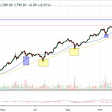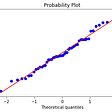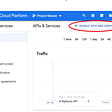# Fitting a log-normal distribution

Hello,

I’m trying to plot a fit to a log-normal distribution. I have the statistics and machine learning toolbox, but I am confused as how to apply the log-normal fit function to this data.

Below is some minimum working code to create a log-normal distribution, but I do not know how to progress further with this fit. The ‘lognfit’ function requires only a 1 dimensional input vector, not the two input parameters I have (i.e. x and p).

]

As an alternative, I’ve also tried fitting using cftool and inputting the log-normal probability distribution function, but unfortunately I did not get a successful fit.

Any advice that you could give would be greatly appreciated.

`% create x datax = (0.01:0.02:11); % define lognormal distribution parameterssigma=1;mu=1;% create a log normal distribution with these parametersy = lognpdf(x,sigma,mu);% plot the resulting distributionfigure, plot(x,y);`

NOTE:-

Matlabsolutions.com provide latest MatLab Homework Help,MatLab Assignment Help for students, engineers and researchers in Multiple Branches like ECE, EEE, CSE, Mechanical, Civil with 100% output.Matlab Code for B.E, B.Tech,M.E,M.Tech, Ph.D. Scholars with 100% privacy guaranteed. Get MATLAB projects with source code for your learning and research.

Your problem is a not uncommon one for people who don’t understand random distributions.

The fact is, you don’t have data as samples from a lognormal distribution. You have points taken as values off the lognormal PDF. You cannot use lognfit to fit that data.

Lets see how to do it, in a way that will work. I’ll start with a simple example, using a normal.

Here, I’ll generate some random samples using randn. The mean should be 2, standard deviation 3. But the estimated values for those parameters will not be exactly those numbers of course since they are estimates, taken from a finite sample.

`X = randn(1000,1)*3 + 2;[MUHAT,SIGMAHAT] = normfit(X)MUHAT =`

--

--

--

## More from Technical Source

Simple! That is me, a simple person. I am passionate about knowledge and reading. That’s why I have decided to write and share a bit of my life and thoughts to.

Love podcasts or audiobooks? Learn on the go with our new app.

## Building a Data Warehouse in Python Using PostgreSQL## Why Data Scientist?## What are QQ Plots?## An Economist also a Data Analyst?## Beginner’s Guide: Hyperparamter Tuning## D4S Sunday Briefing #90## Accessing Google Big Query from Jupyter notebook.## KKBox Churn Prediction Solution## Technical Source

Simple! That is me, a simple person. I am passionate about knowledge and reading. That’s why I have decided to write and share a bit of my life and thoughts to.

## How to draw 2 random number simultaneously which are smaller than 1 together?